# Implementation of Visual Clustering Strategy in Self-Organizing Map for Wear Studies Samples Printed Using FDM

Implementation of Visual Clustering Strategy in Self-Organizing Map for Wear Studies Samples Printed Using FDM

Latchoumi Thamarai Pugazhendhi Raja Kothandaraman Balamurugan Karnan

Department of Computer Science and Engineering, SRM Institute of Science and Technology, Ramapuram Campus, Chennai 600089, Tamil Nadu, India

Department of Mechanical Engineering, VFSTR (Deemed to be University), Guntur 522213, AP, India

Corresponding Author Email:
Page:
531-539
|
DOI:
https://doi.org/10.18280/ts.390215
27 January 2022
|
Accepted:
17 March 2022
|
Published:
30 April 2022
| Citation

OPEN ACCESS

Abstract:

In general, visual clusters are preferred over large data sets; this is an attempt to take advantage of cluster techniques to reduce the mathematical complexity of small data sets. To identify the possibility of implementing the clustering technique in a small dataset, the wear observations of PLA/Cu composite samples printed using the Fused Deposition Model (FDM) is taken into consideration. In this study, the Self Organizing Map (SOM) tool as a non-supervised Neural Network (NN) is used to visualize the data. Here, SOM combinations with vector quantification and projection are used to identify or rank the wear machinability parameters on the new composite filament printed under different FDM conditions. The competitive layer in SOM will classify the given parameters of the wear machine (vectors) at any number of dimensions may be into several groups of layer neurons. The limitation of SOM is map size which cannot exceed 1000 units of training. However, for the small data set under consideration, the extent of these limits will not affect performance. The SOM algorithm developed for the study of wear provides the outlet within the acceptable range. In addition, the linear regression analysis is carried out for the output response to measure the wear characteristics of the machining observation.

Keywords:

fused deposition modeling, linear regression, neural network, self-organizing map, wear study

1. Introduction

FDM is a renowned production method due to its ease of performing the complicated work required in developing the final product. But, according to Mohamed et al.  several machining factors in the FDM effect component creation, which leads to unsatisfactory production. Therefore, before printing the samples, a comprehensive analysis of the various factors involved in the FDM is required. Motaparti et al.  evaluated the mechanical properties of the sample prepared on FDM at various machining settings and found that anisotropic properties are present according to the materials and the machining conditions.

According to Fafenrot et al.  adding metals to polymers reduces the mechanical properties of the composite filament and makes it necessary to examine the composite filament. According to Uddin et al.  reinforced metal powders in the PLA as a medium has gained interest because of their ability to support all instinctual and sophisticated forms. According to Gawande et al.  the abundant quantity of copper particles on earth as natural materials can be synthesized/processed via the chemical route; this attribute makes it a viable material for many engineering fields. Salea et al.  fabricated 3D printed samples with copper reinforcement and sintering to transform copper into copper oxide semiconductors to form a 3D semiconductor that is highly sensitive to light, pressure, and temperature.

According to previous research, the post-machining procedure to improve the quality characteristics of the fused deposition model result cannot be overlooked and some meaningful conversation about such an issue is necessary that included as part of this paper. The determination of the most achievable orientation of FDM printing will greatly reduce the probability of post-machining . The use of machining process in the complex geometry created by FDM will increase the machining flow of the surface roughness model. The linear surface distribution model is developed using a genetic algorithm and computational technique, significantly reducing the post-processing of FDM components . The studies have identified the numerous techniques required to improve the surface characteristics of FDM printed samples. In summary, four identical techniques are proposed: optimization of machining settings, slicing technique, selection of appropriate construction direction, and post-treatment. As it turns out, the post-processing method improves the geometric integrity of objects .

During the post-treatment phase, identical manufactured replicas are exposed to hot acetone vapors, and variations in surface varnish, shore hardness, and size accuracy are examined. The analysis revealed that post-treatment ABS copies with hot acetone vapors improved surface gloss and hardness of the edge while causing minor changes in part dimensions .

Market segmentation is accomplished by categorizing people based on their purchasing behavior. Data mining approaches include sharing data into related subsets and bioinformatics analysis, such as grouping genes with similar expression models. The Neural Clustering program will allow you to choose data, build a network and evaluate its results using a variety of visualization tools. The neurons of the layer are organized in 2D topology, which allows it to reflect the distributions and to approach the structure of the dataset in two dimensions. In the system, the SOM batch methodology is used.

In a SOM, a competitive layer will classify a collection of vectors with any number of dimensions in as many groups as the neurons in the layer. It preprocesses, initializes, and forms datasets in a wide variety of topologies and ranges. The map is then divided into groups as a function of the relationships of the variable. Variables may be attributes of an element or a set of measurements collected at a given time. The cornerstone of mass parallel data processing and large-scale parallel computing is the theory of IN. The NN is a highly nonlinear dynamic system that can be used to grasp intelligent thinking, control activities, and make decisions .

Each sensor node functions in a homogenous or heterogeneous mode and is limited in terms of energy and memory. To collect information only under risk conditions in the sensor network over a large region and a long period, an effective protocol is necessary. In terms of network life and energy use, the proposed protocol exceeds the standard PEGASIS protocol. The weight of the winner and its surrounding neurons in the SOM topology is modified irrespective the input vector. When updating the weight, the neurons most distant and closest to the winning neuron, as well as the winning frequency of each neuron, are discovered and considered. The new SOM calculates learning output using three standard variables that are also utilized in input datasets for later stages. The SOM method family will effectively cover the entire detection area. The base station sends and receives data through related SOM clustered head nodes .

The observed decomposition technique divides the information into a set of foundational type functions derived from the error function. To create fuzzy entropy, the proposed approach combines the fuzzy function. The use of this combination in building vectors reflects the difficulty and irregularity of each functional variable . Examining key elements reduces the size of entity vectors. The results of the proposed technology demonstrate that it properly assesses bearing degradation and detects high-sensitivity defects for a range of bearing defects . The many types of faults and malfunctions in the diagnostic data of the sensors were examined using time series. The SOM algorithm in NN was introduced to demonstrate that it senses errors better than standard NN. In addition, faults are successfully isolated . The SOM learning node selects the neuron closest to the input data as the victor. To change the surrounding neurons, the distance between the input data and itself is used. Compared to the current knowledge discovery system, the fault tolerance level is 92.37% .

The unsupervised Deep SOM design is unique and adheres to the same in-depth learning algorithm principles. This architecture has the potential to be very valuable in a Big Data context for machine learning applications. Experiments have been conducted on how the proposed design achieves this performance . The semi-supervised diagnostic technique for machine fault detection and classification is based on a distance-preserving SOM, which can also be used to directly view SOM learning outcomes. An experimental study on a gearbox and bearings revealed that the proposed technique is effective in identifying early gear pitting failures and categorizing various bearing defects and degrees of ball bearing defects .

The visual clustering technique for machine-part cell creation uses the SOM algorithm and an unsupervised neural network to enhance the efficiency of group technology, the efficiency of cell formation, and the accuracy of SOM. The findings demonstrate that the suggested technique not only produces the best and most accurate answer but also in certain cases, the results obtained are even superior to the previously stated results . Relational Perspective Mapping (RPM) is used to create more realistic images of hyperspectral data. The SOM result is fed into the RPM method, which is a nonlinear dimensionality reduction approach that generates a two-dimensional map from high-dimensional data. RPM provides additional distance information using topologic information provided by the SOM. Consequently, the color scheme accurately represents the local spectral distances of the data between the pixels .

Three comparative simulation experiments and a suggested application to digital innovation data demonstrate the proposed S-resilience SOM and efficacy. Additional documents are available for this purpose . The study proposes hybrid models that use vibration signals in conjunction with SOMs to forecast cutting power and waviness in the circular sawing process of Douglas-fir wood at very high feed speeds. SOM is suggested for automatic functions in machining or monitoring the state of the tool, where the selection of human functions is difficult due to the unpredictability of the process. When SOM is coupled with an ANN or ANFIS, it creates a strong intelligent model for monitoring complicated operations like wood circular sawing .

While the new technology has made recording hyperspectral data cheaper, the acquisition of baseline data (ground truth) remained costly and time-consuming. Methodological techniques that process datasets with much more hyperspectral input data than standard data are needed. Supervised Self-organizing Maps (SuSi) is introduced, which can perform an unsupervised, supervised, and semi-supervised classification, as well as regression on large-scale data .

2. Materials and Methods

A new PLA/Cu composite filament for FDM is successfully fabricated using a hot extrusion process . The fabricated filament is printed to an identified FDM condition to the sample size of 10x10x50mm. FDM conditions like raster angle, infill density is maintained to be as constant as 45o and 100%, respectively . The printed samples are tested using Pin-on-Disc wear tester machine for the varied parameters to Taguchi L27 orthogonal array. The test samples are grounded to fine surface finish through emery paper (80 grit size), then through polish machine using acetone as etchant. New flat surface is formed due to the rubbing action will have a complete contact with the flat disc and it has to be ensured. The test samples are mounted in the fixture and fit perpendicular to the disc. The disc (Counter material) is made of EN 31 steel. The pin is loaded with the counter weight material on the other side by dead weight mechanism, which could keep the pin in contact with the disc. The disc surface is cleaned with the acetone to remove the dust particles. As the disc rotates at specified rpm, the wear occurs in the test sample is measured through height loss method. The wear rate is calculated and the same is expressed in terms of wear loss per unit sliding distance. The FDM machine and the wear tester are shown in Figure 1. The parameters and the levels of the wear test study are illustrated in Table 1. Here, load, track diameter and speed are taken as the independent parameters (machining parameters) and wear rate and the frictional force are taken as the dependent parameters (output responses).

## 1.pngFigure 1. Experimental arrangements for PLA/Copper filament and wear study

Table 1. Wear parametric conditions and the output responses

 Load (N) Diameter (mm) Speed (rpm) Wear Rate (mm/sec) Frictional Force (N) 20 40 400 101.01 06.8 20 40 500 164.98 10.2 20 40 600 214.00 07.6 20 50 400 138.87 08.1 20 50 500 201.01 08.9 20 50 600 229.75 10.7 20 60 400 243.21 09.0 20 60 500 282.64 10.1 20 60 600 356.09 13.6 30 40 400 144.59 10.1 30 40 500 235.03 11.6 30 40 600 283.48 12.5 30 50 400 265.92 12.8 30 50 500 334.37 13.4 30 50 600 388.01 14.1 30 60 400 393.25 13.1 30 60 500 399.01 14.1 30 60 600 536.14 14.7 40 40 400 340.64 14.3 40 40 500 393.09 15.2 40 40 600 466.53 16.9 40 50 400 448.98 16.0 40 50 500 507.42 15.9 40 50 600 585.87 17.7 40 60 400 586.31 17.3 40 60 500 609.75 18.7 40 60 600 601.01 19.1
3. SOM Algorithm

SOM is a combination of vector projection and vector quantification algorithm and it is made up of low-dimensional neurons arranged in a grid. A dimensional weight vector wi = [wi1, wi2, ..., wid] is used to represent each neuron. The size of the input vectors is denoted by d. The topology, or map structure, of neurons, is determined by their connections to neighboring neurons through a neighborhood relationship as shown in Figure 2.

## 2.pngFigure 2. Structure of SOM layer

3.1 Algorithm for sequential training

Step 1: The input dataset is selected from a random sample vector x.

Step 2: The Euclidean distance calculation is used to calculate the distance between all weight vectors.

d=√[(x22–x11)2+(y22–y11)2]

where,

(x11, y11) are the coordinates of one point.

(x22, y22) are the coordinates of the other point.

d is the distance between (x11, y11) and (x22, y22).

Step 3: Using Eq. (1), the neuron with the weight vector closest to the input vector x is called the Best-Matching Unit (BMU).

||$y-w_{c} \|=\min \left\{|| y-w_{t}||\right\}$     (1)

where, ||. || denotes the unit of distance, which is usually the Euclidean distance.

Step 4: The SOM weight vectors are modified after the BMU is found, bringing the BMU closer to the input space.

Step 5: The BMU's topological neighbors are viewed in the same way.

Step 6: The t-weight vector's update rule is shown in Eq. (2):

wt(x+1)=wt(x)+α(x)pct(x)[y(x)-wt(x)]     (2)

The function α(x) is the learning rate, and x indicates time. y(x) is a random input vector of the input dataset. Around the winning unit c, the function pct(x) is a neighborhood Kernel.

Let V and W be vector spaces over a field (or more generally, modules over a ring) and let T be a linear map from V to W. If 0W is the zero vector of W, then the kernel of T is the preimage of the zero subspace {0W}; that is, the subset of V consisting of all those elements of V that are mapped by T to the element 0W. The kernel is usually denoted as ker T, or some variation thereof: Ker $\mathrm{T}=\{\mathrm{v} \in \mathrm{V}: \mathrm{T}(\mathrm{V})=0 \mathrm{w}\}$.

Step 7: Repeat steps 2 to 6 until getting the optimized result.

4. Results and Discussions

4.1 Training wear parameters using SOM

In SOM, fixing the bound limit (Lower Bound (LB) and Upper Bound (UB)) was always a challenging task for the researcher. The significance of changing the levels in the bound considerably affects the performance of the system to the greater effect . So to execute a new task, based upon the required performance of the system and all the demand of need the bound limits can be fixed to the requirements . In this L27 dataset (Table 1), the LB and UB are set as 5 and 10 respectively. The number of training iterations is determined by the initialization and configuration of nodes. As the bound level takes the square of its value to form the grids. Since LB set as 5, it would result to have 25 grids which were shown in Figures 3 and 4.

## 3.png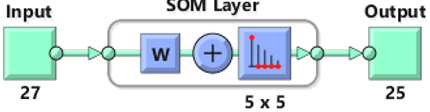Figure 3. LB = 5 training iterations

## 4.png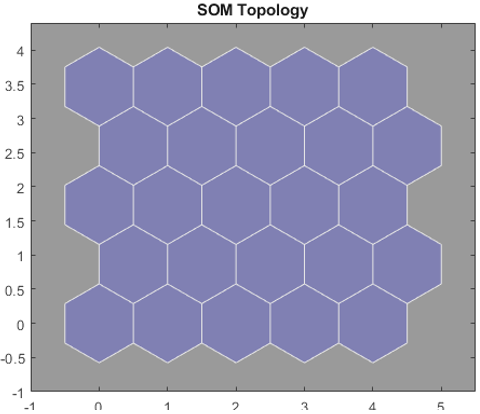Figure 4. SOM topology of 25 grids

The three main steps in the training are: i) choosing an input vector, ii) determining the BMU for the input vector, iii) updating the weight vectors of SOM nodes using parameters like learning rate and neighborhood function. A learning rate is a number that decreases with the increase in the number of iterative steps.

• $\mathrm{p}=5 \sqrt{m}$ is the number of cartographic units, and m is the number of data samples.
• The map is made up of a hexagonal lattice pattern on a rectangular board. The lateral length ratio is the ratio of the two largest eigenvalues of the data covariance matrix.
• The neighborhood function is Gaussian $\mathrm{p}_{\mathrm{ct}}(\mathrm{x})=e^{\frac{\delta_{c t}^{2}}{2 r}}\left(x^{2}\right)$ where, $\delta_{c t}^{2}$ on the map grid, is the distance between the units c and t and calculated the weight.
• The radius of the neighborhood per hour x is r(x). The radius, like the rate of learning, is a monotonously decreasing function of time. The starting radius is determined by the chart's height, but the end radius is always a. The learning rate starts at 0.5 and goes down to (almost) zero.
• Periods are used to calculate the duration of training: each period corresponds to a data transfer. The number of time frames is proportional to the number of map units divided by the number of data samples, or p=m.

4.2 Training wears parameters using SOM for LB 5

The SOM neighboring neuron connections of 25 grids are shown in Figure 5(a). The simulation time is directly proportional to the number of neurons, as it is important to maximize the active neurons while minimizing the number of inactive neurons through simulation time. Figure 5(b) shows the distribution of active and inactive neurons for the LB 5. All yellow color grids represent the active neurons whereas the wide range of orange color represents the inactive neurons. A decrease in the orange color in inactive neurons may have the possibility to enter into the optimized zone. The black color region represents the error message of SOM.

## 5.png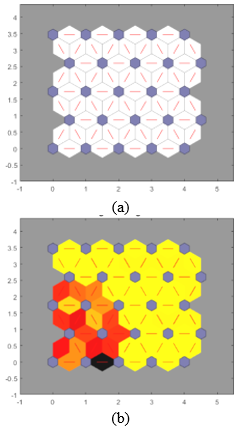Figure 5. (a) SOM neighbor connections of 25 grids; (b) SOM neighbor connections of 25 grids with weight distances of active and inactive neurons between clusters

The SOM architecture design is given to each wear machine condition. Through this for LB 5 that has 25 grids shows the identical clusters for every wear parametric condition. The SOM representation for L27 wears observations as shown in Figure 6. Since the black regions represent the active neurons, the dark-colored grids cannot enter into the neighbor classification irrespective of colors. Pale colors have some possibilities to enter into the neighboring clusters. Based on the micro-level analysis of each experiment, the weight from the input image shown in Figure 6 represents different colors. The micro level error back propagation scheme to train SOM directly based on spiking activities. The rate-coded error is defined and efficiently computed and back-propagated across the microscopic levels. Since the black regions represent the active neurons, the dark-colored grids cannot enter into the neighbor classification irrespective of colors. Each color has its weightage error over the considered value. For example input, 25 figures have 6 color grids (three orange, one brown, one pale brown, one yellow). The severity of the error is represented by the darkness of the color. For instance, here brown and pale brown color is out of classification but has close relation with each to its value. Pale brown can enter into the neighbor cluster and it further confirms the error rate is considerably less than a dark brown grid. Ultimately, the error rate on each observation can be easily identified and can be grouped from Figure 6.

## 61.png## 62.png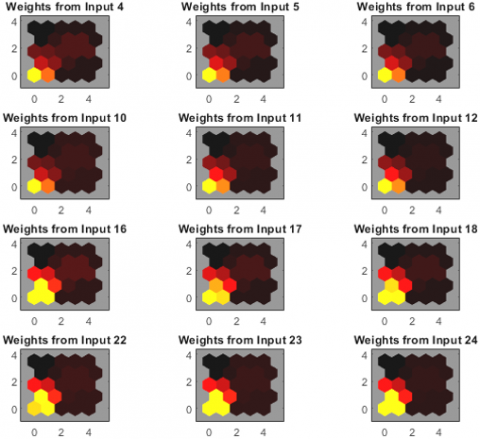Figure 6. SOM neighbor connections of 25 grids for L27 input data with active and inactive neurons

To identify the insignificant grids for LB=5, the hit value examination was conducted for the proposed 25 grids. The training results are shown in Figure 7. Among the available 25 grids, 5 grids show the hit point value and identified insignificant grids. These 5 grids have error values greater than the neighborhood grids and fail to form clusters. The accuracy of clustering for LB 5 can be calculated by Equation 3.

Accuracy rate $=\frac{(\text { Available grids }-\text { Hit grids })}{\text { Available grids }} * 100$     (3)

By using Eq. (1) the rate of accuracy is predicted to 80%. SOM weight positions of active neuron classification are shown in Figure 8. From Figure 8 it was well clear to infer that the given L27 wear observations are being categorized into 25 grids based on the given LB=5 display 10 neuron classification. Due to error difference, the hit grids have a position with their classification and were represented with blue color. The remaining 20 active neuron grids are being classified into 5 groups and displayed as green color. Among the active neuron classification, one set of the group is far away from the remaining active 4 groups is because of the high level of wear machining condition and its output response. This can be verified from Figure 8. To identify the numbers of grids in each active neuron classification can be validated or it can be verified from Figure 6.

## 7.pngFigure 7. SOM neighbor hit connections

## 8.pngFigure 8. SOM weight positions of active neuron classification

4.3 Training wears parameters using SOM for UB=10

In the present study, the UB is set to 10 and there would be 100 grids, as seen in Figures 9 and 10. Figures 11(a) and 11(b) illustrate the SOM adjacent neuron connections of 100 grids and the distribution of active and inactive neurons for the UB 10. The discussion on the color representation of each grid for the UB=10 is similar to LB (please ref. Training wears Parameters using SOM for LB 5). Every situation of the wear machine is provided by the SOM architecture design. As a result, each parametric condition of wear for UB 10, which contains 100 grids, exhibits similar clusters. Figure 12 shows the observations in the SOM representation for L27 for UB=10. The discussion in Figure 12 is similar to LB. Finally, each observation's error rate may be easily determined and aggregated.

## 9.png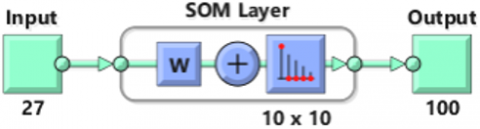Figure 9. UB = 10 training iterations

## 10.png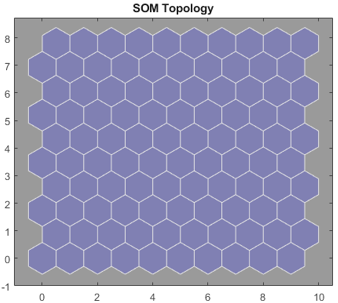Figure 10. SOM topology of 100 grids

## 11.png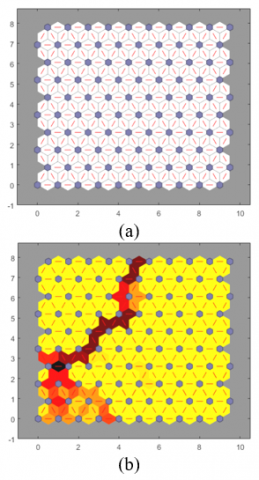Figure 11. (a) SOM neighbor connections of 100 grids; (b) SOM neighbor connections of 100 grids with weight distances of active and inactive neurons between clusters

## 12.png## 122.png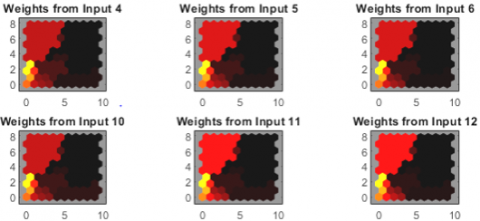## 123.pngFigure 12. SOM neighbor connections of 100 grids for L27 input data with active and inactive neurons

The assessment of the impact value for the predicted 100 grids was undertaken to determine the grids without consequence for UB=10. Figure 13 shows the outcomes of the training. Of the 100 available grids, 5 grids display the contact point value and identify non-significant grids. All five grids have higher error values than neighborhood grids, so they are not able to form clusters. Eq. (3) can be used to compute the clustering accuracy for UB=10. The SOM method could effectively discern the result states, and the rate of clustering accuracy could exceed 95%. SOM weight positions of active neuron classification are shown in Figure 14.

## 13.pngFigure 13. SOM neighbor hit connections

## 14.png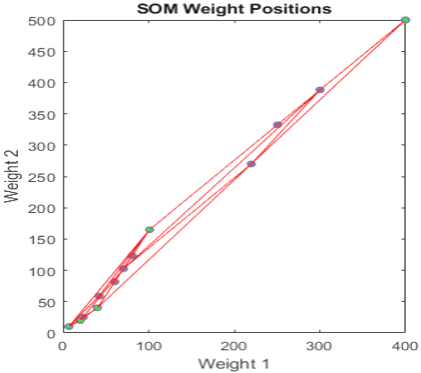Figure 14. SOM weight positions of active neuron classification

Training criteria affect it. Due to the random order of the points, it can produce different solutions for the same data, particularly in 3D for range images with complex boundaries.

A trial exercise is performed for UB=8 to understand the effect of change in the accuracy rate. For the UB=8 (64 grids), 5 hit grids occurred in SOM. Applying for this number in Eq. (3) will yield 92.2% accuracy. In the case of increasing UB beyond 10 increases the hit grid value and progress to have a reduced accuracy rate. For L27 Orthogonal Array-Experimental conditions UB=10 provides an acceptable range of accuracy. Between this range (LB=5 and UB=10) has provided the same hit grid values of 5. Decreasing the LB no change in the grid is observed whereas the adverbs effect was noted for UB.

4.4 Regression analysis for wear rate

Fit model linear regression analysis is conducted for the wear rate observations (Table 2) using Matlab. The significance of each wear parameter is evaluated through the ANOVA table. Through analysis, it is proven that the applied load provides greater wear on the machined sample when compared to speed and track diameter with a contribution of 62%. The track diameter shows the significant effect of 25% followed by the speed with 9% of the contribution. From the model summary, residual squares and adjacent residual squares are greater than 95% close to the acceptable range of tolerance. The linear regression Equation 4 developed through this model is shown below. From the normal probability, the plot was observed that all the experiments are linearly fit with one other shown in Figure 15.

Table 2. Fit model linear regression analysis for wear rate observations

 Analysis of variance source DF Adj_MS Adj_SS Contribution Regression 6 98552.9 591317 96.70% Load (N) 1 1518.1 1518 61.80% Diameter (mm) 1 2707.6 2708 25.16% Speed (rpm) 1 4186.6 4187 9.05% Load (N)*Diameter (mm) 1 3164.2 3164 0.52% Load (N)*Speed (rpm) 1 128.5 129 0.02% Diameter (mm)*Speed (rpm) 1 959.4 959 0.16% Error 20 3.30% Total 26 100.00% Model Summary R-sq=96.70%, R-sq(adj)= 95.71%

Wear Rate (mm/sec) = -853 + 8.01 Load + 8.84 Diameter + 1.100 Speed + 0.1624 Load * Diameter - 0.00327 Load * Speed - 0.00894 Diameter * Speed      (4)

## 15.png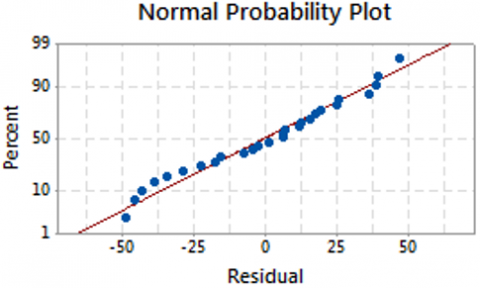Figure 15. Residual plots for wear rate

4.5 Regression analysis for frictional force

Regression study is conducted for frictional force, from the ANOVA observation from Table 3, load shows the significant contribution of 78% followed by track diameter and speed of 11% and 6% respectively. Residual squares and adjacent residual squares show the consistency of 95% was acceptable. The regression equation frictional force is shown as Equation 3. From the normal probability plot, three observations show inconsistency fit with the linear model which could be measured through Residual R2 value in Figure 16. These 3 variations are occurred due to the improper dispersion of copper particles while printing the sample. Further study is required to explore the reason for the deviation in observations.

Table 3. Fit model linear regression analysis for Frictional Force observations

 Analysis of variance source DF Adj_MS Adj_SS Contribution Regression 6 49.6207 297.724 95.48% Load (N) 1 4.2056 4.206 77.85% Diameter (mm) 1 0.0300 0.030 10.69% Speed (rpm) 1 0.1401 0.140 6.71% Load (N)*Diameter (mm) 1 0.0300 0.030 0.01% Load (N)*Speed (rpm) 1 0.3008 0.301 0.10% Diameter (mm)*Speed (rpm) 1 0.4033 0.403 0.13% Error 20 4.52% Total 26 100.00% Model Summary R-sq=95.48%, R-sq(adj)=94.13%

Frictional Force (N) = -7.20 + 0.421 Load + 0.029 Diameter + 0.0064 Speed + 0.00050 Load * Diameter - 0.000158 Load * Speed + 0.000183 Diameter * Speed      (5)

## 16.png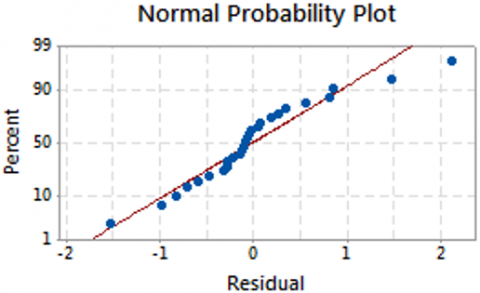Figure 16. Residual plots for frictional force

From the regression analysis, it is confirmed that for both the considered output responses (wear rate and frictional force) the load plays the major contribution. Due to the increase in the load the wear rate and the frictional force gets increased drastically when compared to the other two considered parameters (track diameter and speed). The wear rate of FDM printed PLA/Copper samples was greatly limited because of the reinforced copper particle. At wear operating conditions, the thermal energy was dissipated through the sample. This energy loses the PLA structure and avoids the slipping of copper particles. When compared to other metals copper tends to wear hence the energy observed by the copper is transformed into two phases (Copper wear on its particles ii) high heat transfer to the neighborhood particles). If the wear region posses the excess copper particle may lead to wear resistance and temperature transfer among which directly signifies the output parameters. An adverse effect is observed in the absence of copper particles in the wear sample. The uniform distribution of the copper particle on the FDM printed sample may not be possible irrespective of any technique adopted while preparing the sample. This action may greatly influence the output parameter which significantly disturbs the machining condition while performing the classification for the machining parameter like SOM.

5. Conclusion

An attempt has been made by implementing this SOM technique in NN for wear study to analyze and to provide customized algorithms which were expected to work for this small dataset effectively. The SOM inherent characteristics enable it to detect the grid orientation and sub-boundaries automatically (visual mode only). SOM when compared with other parameterization methods, SOM involves all sample points for grid creation, not only considering boundary points. SOM recognizes the grid orientation as well as the four sub-boundaries locations. SOM does not require any previous network structure decisions, such as the number of nodes or grid in the output layer and it reduces the search and computational time and cost. The proposed system condition for the given LB and UB produces an accuracy of 80% and 95% respectively. Change in the level of UB will change the hit grid value and retain the accuracy level as UB=10. For any system having a small dataset (<30 observations) the proposed LB=5 and UB=10 will be sufficient to get an acceptable level of accuracy rate.

References

 Mohamed, O.A., Masood, S.H., Bhowmik, J.L. (2016). Experimental investigations of process parameters influence on rheological behavior and dynamic mechanical properties of FDM manufactured parts. Materials and Manufacturing Processes, 31(15): 1983-1994. https://doi.org/10.1080/10426914.2015.1127955

 Motaparti, K.P., Taylor, G., Leu, M.C., Chandrashekhara, K., Castle, J., Matlack, M. (2017). Experimental investigation of effects of build parameters on flexural properties in fused deposition modelling parts. Virtual and Physical Prototyping, 12(3): 207-220. http://dx.doi.org/10.1080/17452759.2017.1314117

 Fafenrot, S., Grimmelsmann, N., Wortmann, M., Ehrmann, A. (2017). Three-dimensional (3D) printing of polymer-metal hybrid materials by fused deposition modeling. Materials, 10(10): 1199. http://dx.doi.org/10.3390/ma10101199

 Uddin, S.M., Mahmud, T., Wolf, C., et al. (2010). Effect of size and shape of metal particles to improve hardness and electrical properties of carbon nanotube reinforced copper and copper alloy composites. Composites Science and Technology, 70(16): 2253-2257. https://doi.org/10.1016/j.compscitech.2010.07.012

 Gawande, M.B., Goswami, A., Felpin, F.X., et al. (2016). Cu and Cu-based nanoparticles: Synthesis and applications in catalysis. Chemical Reviews, 116(6): 3722-3811. https://doi.org/10.1021/acs.chemrev.5b00482

 Salea, A., Prathumwan, R., Junpha, J., Subannajui, K. (2017). Metal oxide semiconductor 3D printing: preparation of copper (II) oxide by fused deposition modelling for multi-functional semiconducting applications. Journal of Materials Chemistry C, 5(19): 4614-4620. https://doi.org/10.1039/c7tc00990a

 Ahn, D.K., Kim, H.C., Lee, S.H. (2005). Determination of fabrication direction to minimize post-machining in FDM by prediction of non-linear roughness characteristics. Journal of Mechanical Science and Technology, 19(1): 144-155. https://doi.org/10.1007/BF02916113

 Ahn, D., Kim, H., Lee, S. (2007). Fabrication direction optimization to minimize post-machining in layered manufacturing. International Journal of Machine Tools and Manufacture, 47(3-4): 593-606. https://doi.org/10.1016/j.ijmachtools.2006.05.004

 Bual, G.S., Kumar, P. (2014). Methods to improve surface finish of parts produced by fused deposition modeling. Manufacturing Science and Technology, 2(3): 51-55.

 Kalyan, K., Singh, J., Phull, G.S., Soni, S., Singh, H., Kaur, G. (2018). Integration of FDM and vapor smoothing process: Analyzing properties of fabricated ABS replicas. Materials Today: Proceedings, 5(14): 27902-27911. https://doi.org/10.1016/j.matpr.2018.10.029

 Li, W., Zhang, S., He, G. (2013). Semisupervised distance-preserving self-organizing map for machine-defect detection and classification. IEEE Transactions on Instrumentation and Measurement, 62(5): 869-879. https://doi.org/10.1109/TIM.2013.2245180

 Chattopadhyay, M., Dan, P.K., Mazumdar, S. (2012). Application of visual clustering properties of self organizing map in machine–part cell formation. Applied Soft Computing, 12(2): 600-610. https://doi.org/10.1016/j.asoc.2011.11.004

 Gardner, W., Maliki, R., Cutts, S.M., Muir, B.W., Ballabio, D., Winkler, D.A., Pigram, P.J. (2020). Self-organizing map and relational perspective mapping for the accurate visualization of high-dimensional hyperspectral data. Analytical Chemistry, 92(15): 10450-10459. https://doi.org/10.1021/acs.analchem.0c00986

 Riese, F.M., Keller, S., Hinz, S. (2019). Supervised and semi-supervised self-organizing maps for regression and classification focusing on hyperspectral data. Remote Sensing, 12(1): 7. https://doi.org/10.3390/rs12010007

 Sagnika, S., Mishra, B.S.P., Meher, S.K. (2020). Improved method of word embedding for efficient analysis of human sentiments. Multimedia Tools and Applications, 79(43): 32389-32413. https://doi.org/10.1007/s11042-020-09632-9

 Nasir, V., Cool, J. (2020). Intelligent wood machining monitoring using vibration signals combined with self-organizing maps for automatic feature selection. The International Journal of Advanced Manufacturing Technology, 108(5): 1811-1825. https://doi.org/10.1007/s00170-020-05505-5

 Sakkari, M., Zaied, M. (2020). A convolutional deep self-organizing map feature extraction for machine learning. Multimedia Tools and Applications, 79(27): 19451-19470. https://doi.org/10.1007/s11042-020-08822-9

 Vesanto, J. (2000). Neural network tool for data mining: SOM toolbox. In Proceedings of symposium on tool environments and development methods for intelligent systems (TOOLMET2000), Oulu, Finland, pp. 184-196.

 Ahmed, M.T., Setu, B.S., Rahman, M., Islam, A.T. (2020). Development of SOM neural network based energy efficient clustering hierarchical protocol for wireless sensor network. International Journal of Advanced Smart Sensor Network Systems (IJASSN), 10(1/2/3). https://doi.org/10.5121/ijassn.2020.10301

 Zair, M., Rahmoune, C., Benazzouz, D. (2019). Multi-fault diagnosis of rolling bearing using fuzzy entropy of empirical mode decomposition, principal component analysis, and SOM neural network. Proceedings of the Institution of Mechanical Engineers, Part C: Journal of Mechanical Engineering Science, 233(9): 3317-3328. https://doi.org/10.1177/0954406218805510

 Yang, J., Guo, Y., Zhao, W. (2019). Long short-term memory neural network based fault detection and isolation for electro-mechanical actuators. Neurocomputing, 360: 85-96. https://doi.org/10.1016/j.neucom.2019.06.029

 Ibrahim, L.M., Basheer, D.T., Mahmod, M.S. (2013). A comparison study for intrusion database (Kdd99, Nsl-Kdd) based on self organization map (SOM) artificial neural network. Journal of Engineering Science and Technology, 8(1): 107-119.

 Yao, X.Q., Tang, G., Hu, X. (2018). Method for recognizing mechanical status of container crane motor based on SOM neural network. IOP Conference Series: Materials Science and Engineering, 435(1): 012009. https://doi.org/10.1088/1757-899X/435/1/012009

 Pavan, M.V., Balamurugan, K., Balamurugan, P. (2020). Compressive test fractured surface analysis on PLA-Cu composite filament printed at different FDM conditions. IOP Conference Series: Materials Science and Engineering, 988(1): 012019. https://doi.org/10.1088/1757-899X/988/1/012019

 Pavan, V.M., Balamurugan, K., Latchoumi, T.P. (2021). PLA-Cu reinforced composite filament: Preparation and flexural property printed at different machining conditions. Advanced Composite Materials, 31(1): 102-117. https://doi.org/10.1080/09243046.2021.1918608

 Baptista, M.L., Henriques, E.M., Goebel, K. (2021). A self-organizing map and a normalizing multi-layer perceptron approach to baselining in prognostics under dynamic regimes. Neurocomputing, 456: 268-287. https://doi.org/10.1016/j.neucom.2021.05.031

 Brito, L.C., da Silva, M.B., Duarte, M.A.V. (2021). Identification of cutting tool wear condition in turning using self-organizing map trained with imbalanced data. Journal of Intelligent Manufacturing, 32(1): 127-140. https://doi.org/10.1007/s10845-020-01564-3